Distance Metrics

It is often useful in image processing to be able to calculate the distance between two pixels in an image, but this is not as straightforward as it seems. The presence of the pixel grid makes several so-called distance metrics possible which often give different answers to each other for the distance between the same pair of points. We consider the three most important ones.

Euclidean Distance

This is the familiar straight line distance that most people are familiar with. If the two pixels that we are considering have coordinates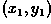and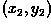, then the Euclidean distance is given by: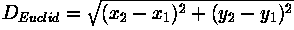City Block Distance

Also known as the Manhattan distance. This metric assumes that in going from one pixel to the other it is only possible to travel directly along pixel grid lines. Diagonal moves are not allowed. Therefore the `city block' distance is given by: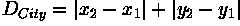Chessboard Distance

This metric assumes that you can make moves on the pixel grid as if you were a King making moves in chess, i.e. a diagonal move counts the same as a horizontal move. This means that the metric is given by: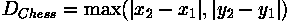Note that the last two metrics are usually much faster to compute than the Euclidean metric and so are sometimes used where speed is critical but accuracy is not too important.

Dithering

Dithering is an image display technique that is useful for overcoming limited display resources. The word dither refers to a random or semi-random perturbation of the pixel values.
Two applications of this techniques are particularly useful: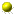Low quantization display: When images are quantized to a few bits (e.g. 3) then only a limited number of graylevels are used in the display of the image. If the scene is smoothly shaded, then the image display will generate rather distinct boundaries around the edges of image regions when the original scene intensity moves from one quantization level to the next. To eliminate this effect, one dithering technique adds random noise (with a small range of values) to the input signal before quantization into the output range. This randomizes the quantization of the pixels at the original quantization boundary, and thus pixels make a more gradual transition from neighborhoods containing 100% of the first quantization level to neighborhoods containing 100% of the second quantization level.Limited color display: When fewer colors are able to be displayed (e.g. 256) than are present in the input image (e.g. 24 bit color), then patterns of adjacent pixels are used to simulate the appearance of the unrepresented colors.

Edge Detectors

Edges are places in the image with strong intensity contrast. Since edges often occur at image locations representing object boundaries, edge detection is extensively used in image segmentation when we want to divide the image into areas corresponding to different objects. Representing an image by its edges has the further advantage that the amount of data is reduced significantly while retaining most of the image information.
Since edges consist of mainly high frequencies, we can, in theory, detect edges by applying a highpass frequency filter in the Fourier domain or by convolving the image with an appropriate kernel in the spatial domain. In practice, edge detection is performed in the spatial domain, because it is computationally less expensive and often yields better results.
Since edges correspond to strong illumination gradients, we can highlight them by calculating the derivatives of the image. This is illustrated for the one-dimensional case in Figure 1.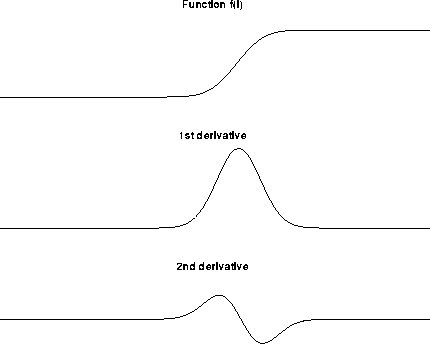Figure 1 1st and 2nd derivative of an edge illustrated in one dimension.

We can see that the position of the edge can be estimated with the maximum of the 1st derivative or with the zero-crossing of the 2nd derivative. Therefore we want to find a technique to calculate the derivative of a two-dimensional image. For a discrete one-dimensional function f(i), the first derivative can be approximated by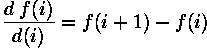Calculating this formula is equivalent to convolving the function with [-1 1]. Similarly the 2nd derivative can be estimated by convolving f(i) with [1 -2 1].
Different edge detection kernels which are based on the above formula enable us to calculate either the 1st or the 2nd derivative of a two-dimensional image. There are two common approaches to estimate the 1st derivative in a two-dimensional image, Prewitt compass edge detection and gradient edge detection.
Prewitt compass edge detection involves convolving the image with a set of (usually 8) kernels, each of which is sensitive to a different edge orientation. The kernel producing the maximum response at a pixel location determines the edge magnitude and orientation. Different sets of kernels might be used: examples include Prewitt, Sobel, Kirsch and Robinson kernels.
Gradient edge detection is the second and more widely used technique. Here, the image is convolved with only two kernels, one estimating the gradient in the x-direction, Gx, the other the gradient in the y-direction, Gy. The absolute gradient magnitude is then given by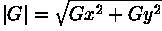and is often approximated withIn many implementations, the gradient magnitude is the only output of a gradient edge detector, however the edge orientation might be calculated with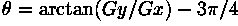The most common kernels used for the gradient edge detector are the Sobel, Roberts Cross and Prewitt operators.
After having calculated the magnitude of the 1st derivative, we now have to identify those pixels corresponding to an edge. The easiest way is to threshold the gradient image, assuming that all pixels having a local gradient above the threshold must represent an edge. An alternative technique is to look for local maxima in the gradient image, thus producing one pixel wide edges. A more sophisticated technique is used by the Canny edge detector. It first applies a gradient edge detector to the image and then finds the edge pixels using non-maximal suppression and hysteresis tracking.
An operator based on the 2nd derivative of an image is the Marr edge detector, also known as zero crossing detector. Here, the 2nd derivative is calculated using a Laplacian of Gaussian (LoG) filter. The Laplacian has the advantage that it is an isotropic measure of the 2nd derivative of an image, i.e. the edge magnitude is obtained independently from the edge orientation by convolving the image with only one kernel. The edge positions are then given by the zero-crossings in the LoG image. The scale of the edges which are to be detected can be controlled by changing the variance of the Gaussian.
A general problem for edge detection is its sensitivity to noise, the reason being that calculating the derivative in the spatial domain corresponds to accentuating high frequencies and hence magnifying noise. This problem is addressed in the Canny and Marr operators by convolving the image with a smoothing operator (Gaussian) before calculating the derivative.

Isotropic Operators

An isotropic operator in an image processing context is one which applies equally well in all directions in an image, with no particular sensitivity or bias towards one particular set of directions (e.g. compass directions). A typical example is the zero crossing edge detector which responds equally well to edges in any orientation. Another example is Gaussian smoothing . It should be borne in mind that although an operator might be isotropic in theory, the actual implementation of it for use on a discrete pixel grid may not be perfectly isotropic. An example of this is a Gaussian smoothing filter with very small standard deviation on a square grid.

Kernel

A kernel is a (usually) smallish matrix of numbers that is used in image convolutions. Differently sized kernels containing different patterns of numbers give rise to different results under convolution. For instance, Figure 1 shows a 3×3 kernel that implements a mean filter.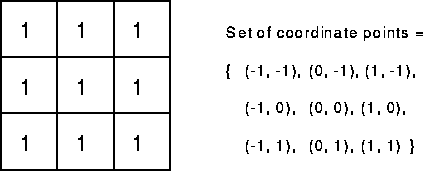Figure 1 Convolution kernel for a mean filter with 3×3 neighborhood.

The word `kernel' is also commonly used as a synonym for `structuring element', which is a similar object used in mathematical morphology. A structuring element differs from a kernel in that it also has a specified origin . This sense of the word `kernel' is not used in HIPR.

Non-linear Filtering

Suppose that an image processing operator F acting on two input images A and B produces output images C and D respectively. If the operator F is linear, then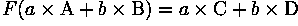where a and b are constants. In practice, this means that each pixel in the output of a linear operator is the weighted sum of a set of pixels in the input image.
By contrast, non-linear operators are all the other operators. For example, the threshold operator is non-linear, because individually, corresponding pixels in the two images A and B may be below the threshold, whereas the pixel obtained by adding A and B may be above threshold. Similarly, an absolute value operation is non-linear: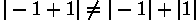as is the exponential operator: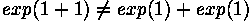Spatial Domain

For simplicity, assume that the image I being considered is formed by projection from scene S (which might be a two- or three-dimensional scene, etc.).
The spatial domain is the normal image space, in which a change in position in I directly projects to a change in position in S. Distances in I (in pixels) correspond to real distances (e.g. in meters) in S.
This concept is used most often when discussing the frequency with which image values change, that is, over how many pixels does a cycle of periodically repeating intensity variations occur. One would refer to the number of pixels over which a pattern repeats (its periodicity) in the spatial domain.
In most cases, the Fourier Transform will be used to convert images from the spatial domain into the frequency domain.
A related term used in this context is spatial frequency, which refers to the (inverse of the) periodicity with which the image intensity values change. Image features with high spatial frequency (such as edges) are those that change greatly in intensity over short image distances.
Another term used in this context is spatial derivative , which refers to how much the image intensity values change per change in image position.

Wrapping and Saturation

If an image is represented in a byte or integer pixel format, the maximum pixel value is limited by the number of bits used for the representation, e.g. the pixel values of a 8-bit image are limited to 255.
However, many image processing operations produce output values which are likely to exceed the given maximum value. In such cases, we have to decide how to handle this pixel overflow.
One possibility is to wrap around the overflowing pixel values. This means that if a value is greater than the possible maximum, we subtract the pixel value range so that the value starts again from the possible minimum value. Figure 1 shows the mapping function for wrapping the output values of some operation into an 8-bit format.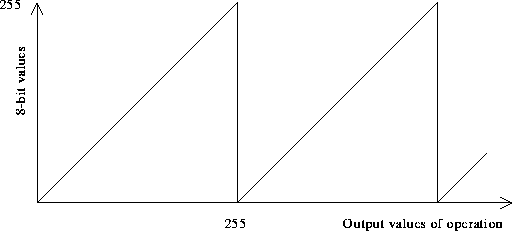Figure 1 Mapping function for wrapping the pixel values of an 8-bit image.

Another possibility is to set all overflowing pixels to the maximum possible values --- an effect known as saturation. The corresponding mapping function for an 8-bit image can be seen in Figure 2.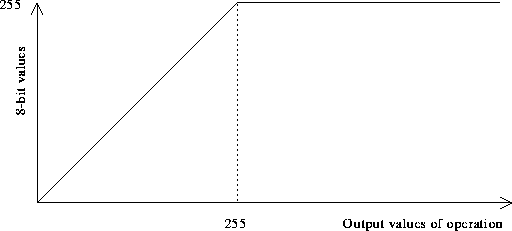Figure 2 Mapping function for saturating an 8-bit image.

If only a few pixels in the image exceed the maximum value it is often better to apply the latter technique, especially if we use the image for display purposes. However, by setting all overflowing pixels to the same value we lose an essential amount of information. In the worst case, when all pixels exceed the maximum value, this would lead to an image of constant pixel values. Wrapping around overflowing pixel retains the differences between values. On the other hand, it might cause the problem that pixel values passing the maximum `jump' from the maximum to the minimum value. Examples for both techniques can be seen in the worksheets of various point operators.
If possible, it is easiest to change the image format, for example to float format, so that all pixel values can be represented. However, we should keep in mind that this implies an increase in processing time and memory.